## List tables by the number of rows in popular relational databases

Queries below list tables by the number of rows in popular relational databases: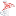SQL Server: List tables by the number of rows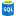Azure SQL Database: List tables by the number of rows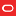Oracle database: List tables by the number of rows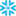Snowflake: List tables by the number of rows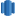Amazon Redshift: List tables by the number of rows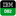IBM Db2: List tables by the number of rows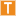Teradata: List tables by the number of rows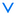Vertica: List tables by the number of rows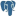PostgreSQL: List tables by the number of rows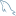MySQL: List tables by the number of rows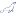MariaDB: List tables by the number of rows
0